Guide to K-Means Clustering with Java - Stack Abuse

# Guide to K-Means Clustering with Java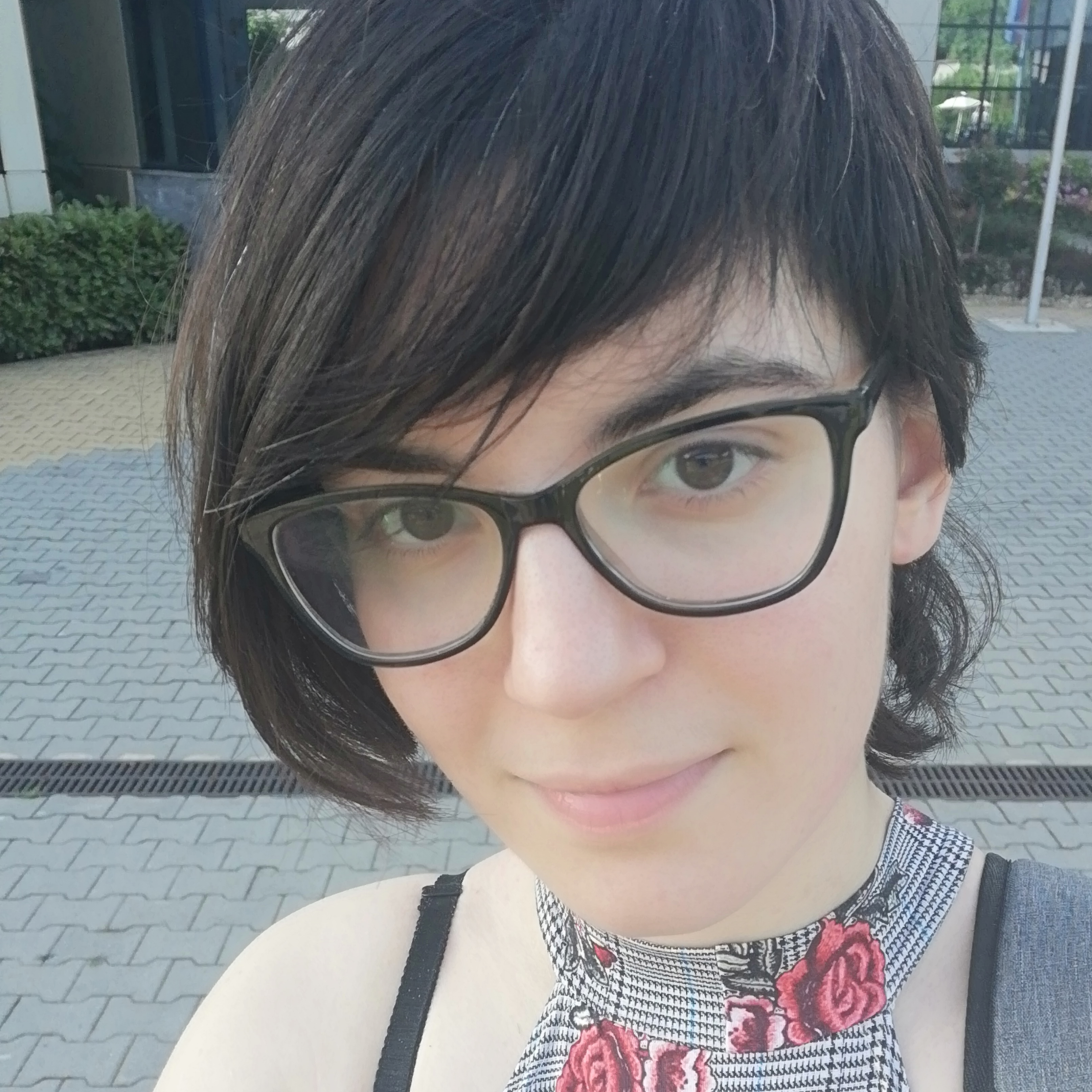### Introduction

K-Means is one of the simplest and most popular clustering algorithms in data science. It divides data based on its proximity to one of the K so-called centroids - data points that are the mean of all of the observations in the cluster. An observation is a single record of data of a specific format.

This guide will cover the definition and purpose of clustering in general what the basic structure of the K-Means algorithm is, what common problems arise when using it and how to handle them, as well as some variations of the algorithm or similar algorithms that will be referenced.

### What is Clustering?

Clustering is the division of data into groups which are meaningful or useful. They can be both, but they can also be only one of those two. Humans naturally cluster objects they perceive into groups and then classify new objects they encounter into one of said clusters.

As a kid, you realize there's such a thing as a tree. You understand the concept of a tree through seeing shared characteristics of trees, as well as dissimilarities of trees from other things. For example, something that has a trunk, branches, and leaves may in general constitute a tree, so things that are similar according to those attributes are perceived by you as trees. They're also dissimilar from non-tree things, like bushes or fungi, because they differ in certain characteristics.

As a kid, you (probably) didn't create an entire taxonomy of the living world around you in order to learn to tell apart a dog from a tree. You did it through clustering. Gradually, as you were exposed to the world, you realized you're seeing certain similarities that can be used to cluster objects together because they will look and behave similarly every time they're encountered.

Using that knowledge about the existence of a meaningful group of data to then recognize new objects is called classification.

Meaningful clustering can help us understand and communicate about the world around us by grouping together things based on their natural structure.

For instance, creating taxonomies of the living world helps us communicate about biology and all of its disciplines and enables us to draw meaningful conclusions, despite it not always being perfectly clear where the lines should be drawn.

Clustering pages on the world wide web according to their topic or content helps search engines recommend us things related to our queries or our interests.

Meaningful clusters are essential for the studies of biology, climate, medicine, business, etc.

Useful clusters are not necessarily reflective of a real world structure or grouping, but rather useful abstractions. They can be used to reduce dimensionality of data by summarizing multiple related attributes into one, it can be used for data compression by creating a prototype table and assigning each prototype an integer to be used as a short-hand for it, as well as to improve performance of some classification algorithms like Nearest Neighbor.

A prototype is a representative data point and it can be one of the observations or just a possible value for an observation. In case of K-Means, the prototype is the mean of all of the observations in the cluster, which is where it derives its name.

### K-Means Algorithm

K-Means is a prototype based clustering algorithm, meaning that its goal is to assign all observations to their nearest prototype.

#### Pseudocode

1. Select K initial centroids
REPEAT:
2. Form K clusters by assigning each observation to its nearest centroid's cluster
3. Recompute centroids for each cluster
UNTIL centroids do not change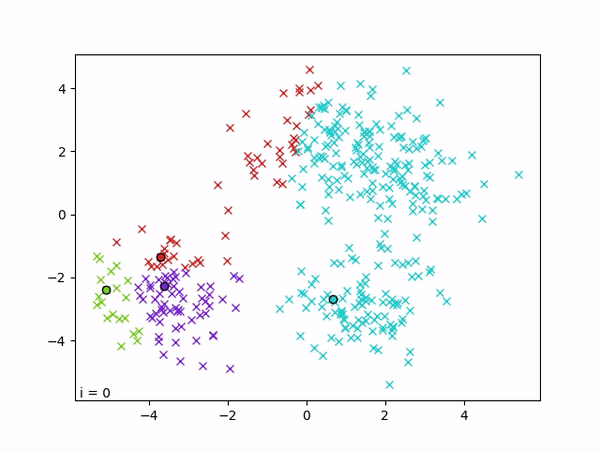#### K-Means Algorithm Explained

The user specifies a number K and the algorithm starts by selecting K observations from the dataset. This selection can be performed in different ways and can greatly influence the end outcome, but for now just imagine randomly selecting K points from the dataset. Let's call those points centroids of clusters.

The next step is to go through all the observations and assort them into clusters. For each observation, its assigned cluster is the same as the one of its closest centroid. If a point is equally close to two centroids, it can be randomly assigned to one of them.

To make this step unbiased, we have to normalize or standardize the data first before applying the algorithm. If we don't, attributes with a wider distribution will have more weight in the classification and we may have even more problems with outliers or otherwise extreme data points than we normally would.

After we've sorted all of the data points into clusters, we recompute centroids for each cluster. We do this by calculating the mean value of all of the variables and we call the result of that operation the new centroid. After creating the new centroid, we repeat the assortment process described above.

It's important to note that in order to calculate a mean value we have to be dealing with quantitative data. If we have qualitative (nominal or ordinal) data, we have to use a different variation of the algorithm (K-Medoid, K-Median, etc) or a combination of different methods depending on the attribute type.

Additionally, if we have a specific goal in mind and depending on the distance measure used in the algorithm, the method of choosing the new centroids can be designed specifically for our use-case and may still be called K-Means, though such cases are rare.

In the most basic case, our stopping criterion would be that every observation's assigned cluster doesn't change from one iteration to the next. Sometimes, we can stop early if the number of observations whose clusters changed is small enough or if the difference in SSE (Sum of Squared Errors) is smaller than a certain threshold.

We usually measure the quality of our clustering by creating an objective function. For K-Means, this objective functions is often aforementioned SSE (Sum of Squared Errors). As its name would imply, SSE is a sum of distances of every observation from its nearest centroid. Thus, our goal when clustering is to minimize SSE:

$$SSE = \sum\limits_{i=1}^K \sum\limits_{j=1}^{\text{cluster size}} d((centroid)_i, (instance)_j)^2$$

#### Choosing Initial Centroids

The easiest way to choose initial centroids is to just pick a number K and pick K random points. However, K-Means is extremely sensitive to the initial pick of centroids and will sometimes output completely different results depending on it. To figure out a more optimal arrangement, we need to solve two problems:

1. How to pick K
2. How to pick K initial centroids

There are several ways of determining the number K:

The approach we'll highlight in detail, because it's commonly used in practice, is the Elbow method. Variance is an expectation of how far away a piece of data will stray away from the mean.

If we take the ratio of variance of centroids and variance of each data point (their expected distances from the mean of all data), for a good clustering, we'll get something close to 1. However, if it gets too close to 1 that may mean that we're overfitting the data - making our model perform perfectly on the given data, but not reflect reality as well.

That's why we use something called the Elbow method. We run the K-Means algorithm with different values of K and plot them on a graph against the aforementioned ratio we get at the end for each of them. The value of K we pick is the one where the "elbow" of the curve is, aka where we start getting diminishing returns as we increase K:Once we have decided on K, we need to pick K starting centroids. Picking this optimally is an NP-hard problem, so an algorithm to approximate a good solution was developed. Let's look at some animations of what could happen if we picked these poorly: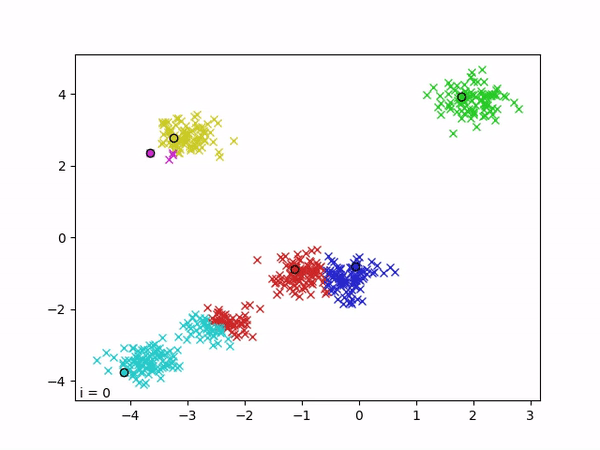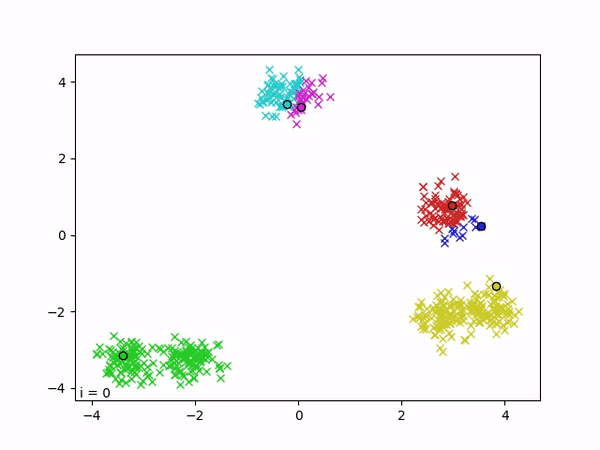One of the algorithms that approximately resolves this problem is called K-Means++. It consists of the following steps:

1. Choose one centroid at random from data points in the dataset, with uniform probability (all points are equally likely to be chosen).
2. For each data point x not chosen yet, compute the distance D(x) from its nearest centroid.
3. Choose one new data point y at random as a new centroid, using weighed probability where y is chosen with the probability of the squared distance .(D(y)*D(y)). In other words, the further away y is from its nearest centroid, the higher the likelihood that it is chosen.
4. Repeat steps 2 and 3 until K centroids have been chosen.
5. Run standard K-Means with centroids initialized.

#### Time and Space Complexity

The time required for K-Means is O(I·K·m·n), where:

• I is the number of iterations required for convergence
• K is the number of clusters we're forming
• m is the number of attributes
• n is the number of observations

This makes sense, because for each iteration O(I), we have to go through all observations O(n) and compute their distance O(m) from each centroid O(K).

Space complexity is O(m·(n+K)) because we're saving n points from our dataset plus the K points for centroids, each point having m attributes.

#### K-Means Implementation in Java

Because of its lack of commonplace support for datasets and data mining, it's not straightforward to implement K-Means in Core Java. You can find the full working code here, but we'll provide a short documentation of the helper class, DataSet, and the implementation of the algorithm itself:

• Class DataSet

## Git Essentials

Check out this hands-on, practical guide to learning Git, with best-practices and industry-accepted standards. Stop Googling Git commands and actually learn it!

• Class Record - a nested class, contains HashMap<String, Double> which stores one row of a table with the key corresponding to the attribute name and value corresponding to its, well, value.
• Fields:
• attrNames - list of attribute names
• records - a list of Records
• minimums and maximums - minimums and maximums for each attribute to be used to generate a random value between them.
• indicesOfCentroids - a list of cluster centroids.
• DataSet(String csvFileName) throws IOException - constructor, reads the data from the provided .csv file and initializes class fields with it.
• HashMap<String, Double> calculateCentroid(int clusterNo) - recomputes a centroid for a given cluster.
• LinkedList<HashMap<String,Double>> recomputeCentroids(int K) - recomputes all K centroids.
• HashMap<String, Double> randomFromDataSet() - returns a random data point out of all of the available data points from the dataset (we need it to initiate the first centroid).
• public HashMap<String,Double> calculateWeighedCentroid() - calculates the distance of all points from currently chosen centroids and weighs them all according to that distance, so the one furthest away is the most likely to be picked, and then picks one of them using roulette selection...)
• static Double euclideanDistance(HashMap<String, Double> a, HashMap<String, Double> b) - calculates the distance between two data points.
• Double calculateTotalSSE(LinkedList<HashMap<String,Double>> centroids) - calculates SSE of all clusters.

The class has some more helper methods, but this should be enough to help us understand the main algorithm.

Now, let's go ahead and implement K-Means, using this class as a helper:

public class KMeans {

// Higher precision means earlier termination
// and higher error
static final Double PRECISION = 0.0;

/* K-Means++ implementation, initializes K centroids from data */
static LinkedList<HashMap<String, Double>> kmeanspp(DataSet data, int K) {
LinkedList<HashMap<String,Double>> centroids = new LinkedList<>();

centroids.add(data.randomFromDataSet());

for(int i=1; i<K; i++){
centroids.add(data.calculateWeighedCentroid());
}

return centroids;
}

/* K-Means itself, it takes a dataset and a number K and adds class numbers
* to records in the dataset */
static void kmeans(DataSet data, int K){
// Select K initial centroids
LinkedList<HashMap<String,Double>> centroids = kmeanspp(data, K);

// Initialize Sum of Squared Errors to max, we'll lower it at each iteration
Double SSE = Double.MAX_VALUE;

while (true) {

// Assign observations to centroids
var records = data.getRecords();

// For each record
for(var record : records){
Double minDist = Double.MAX_VALUE;
// Find the centroid at a minimum distance from it and add the record to its cluster
for(int i = 0; i < centroids.size(); i++){
Double dist = DataSet.euclideanDistance(centroids.get(i), record.getRecord());
if(dist < minDist){
minDist = dist;
record.setClusterNo(i);
}
}
}

// Recompute centroids according to new cluster assignments
centroids = data.recomputeCentroids(K);

// Exit condition, SSE changed less than PRECISION parameter
Double newSSE = data.calculateTotalSSE(centroids);
if(SSE-newSSE <= PRECISION){
break;
}
SSE = newSSE;
}
}

public static void main(String[] args) {
try {
// Read data
DataSet data = new DataSet("files/sample.csv");

// Remove prior classification attr if it exists (input any irrelevant attributes)
data.removeAttr("Class");

// Cluster
kmeans(data, 2);

// Output into a csv
data.createCsvOutput("files/sampleClustered.csv");

} catch (IOException e){
e.printStackTrace();
}
}
}


The sample.csv file contains:

A,B
1,3
2,4
1,2
3,4
1,2
2,2
2,1
10,12
14,11
12,14
16,13
1,1
4,4
10,11
15,13
13,12
4,1
4,3
4,5


Running this code results in a new file, sampleClustered.csv, which contains:

A,B,ClusterId
1.0,3.0,1
2.0,4.0,1
1.0,2.0,1
3.0,4.0,1
1.0,2.0,1
2.0,2.0,1
2.0,1.0,1
10.0,12.0,0
14.0,11.0,0
12.0,14.0,0
16.0,13.0,0
1.0,1.0,1
4.0,4.0,1
10.0,11.0,0
15.0,13.0,0
13.0,12.0,0
4.0,1.0,1
4.0,3.0,1
4.0,5.0,1


We have two clusters, 0 and 1 here. And depending on the characteristics of each of these, the algorithm has clustered them into one of these.

### Possible Problems with K-Means

K-Means has both common problems stereotypical for clustering algorithms and ones specific just to K-Means. Let's go over some of the most common ones and how to handle them.

#### Handling Empty Clusters

A problem we may run into is a cluster not being assigned any observations. If this happens, we need some way of choosing the next centroid for that cluster, but we have no observations to average out. There are multiple approaches to this problem.

1. We could just pick one of the points, for example the observation that is furthest away from any of the other centroids. This method is very sensitive to outliers and only recommended if there are none.

2. Alternatively, we could find the cluster with largest SSE and pick a centroid from it. Doing this would effectively split that cluster and reduce overall SSE more than picking some random point.

#### Outliers

Outliers are a problem for K-Means because they significantly pull any centroids they are attributed to towards them, having undue weight in the calculation.

They can cause additional complications with SSE, as they may force suboptimal clusterings just so the centroid would be closer to the outliers. It is generally recommended to eliminate outliers before using K-Means to avoid this problem.

It is, however, important to note that depending on the application you're using the algorithm for, keeping the outliers might be critical. For instance, in data compression you have to cluster every point, including the outliers. In general, we might be interested in outliers for some purposes (very profitable customers, exceptionally healthy individuals, relationships between wing size and mating speed in Drosophila malerkotliana...).

So while the rule of thumb should definitely be removing the outliers, make sure to consider the purpose of your clustering and the dataset you're working on before making the decision.

#### Local Minimums and Reducing SSE With Postprocessing

As is so often the case with these algorithms, K-Means doesn't guarantee optimality. It might end up in a local minimum - the result that could be improved with some tweaking.

We can lower the total SSE by cleverly splitting existing clusters or by adding a new centroid. If we are splitting a cluster, it's good to pick the one with largest SSE, which will often be the one with the largest number of points as well. If we add a new centroid, it's often good to pick the point that's furthest away from all existing centroids.

If we want to decrease the number of clusters afterwards (for instance, so that we would keep exactly K clusters as the result), we can also use two different techniques. We can either:

1. Merge two clusters (usually the smallest ones or those with lowest SSE)
2. Disperse a cluster by removing its centroid and reassigning its members to other clusters.

#### Finding Non-Existent Clusters

K-Means will find K clusters no matter the underlying data. If there's 3 clusters and you've set K to 5, it will find 5 clusters. If there's no clusters whatsoever, it will still find 5 clusters: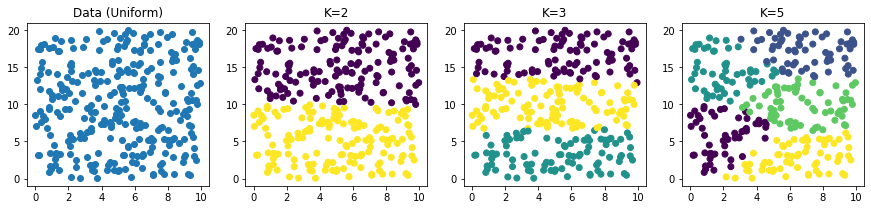There's no way to prevent this in K-Means itself. Instead, one should first check the Hopkin's statistic to see if there are any clusters in the data itself. Hopkin's statistic works by comparing the dataset to a randomly generated uniform set of points.

Say we have our dataset, X, and it has n data points. We sample m of them for analysis.

We then randomly generate another dataset, Y, that follows a uniform distribution. Y also has m data points.

The distance between some member of X and its nearest neighbor, we'll call w.

The distance between some member of Y and its nearest neighbor in X, we'll call u.

The Hopkin's statistic then comes out as:

$$H = \frac{\sum\limits_{i=1}^m u_i}{\sum\limits_{i=1}^m u_i +\sum\limits_{i=1}^m w_i}$$

If our dataset is likely random, the formula will give a number close to .5, while for non-random datasets it will approach 1.

This is because the distances within the set and within the random set will be approximately equal if our set it also random, so we'll get one half.

If it's non-random, distances within the set will be significantly smaller and will contribute negligibly to the denominator, bringing the result closer to 1.

#### Types of Underlying Clusters It Can Recognize

K-Means is very good at recognizing globular clusters of a consistent density and similar size.

This means the cluster will be shaped like a circle, a sphere, or a hypersphere, depending on the dimension you're working in. This is logical, because it relies on the distance from the center to determine whether something belongs to a cluster, so its borders being more or less equidistant from the center naturally makes it spherical: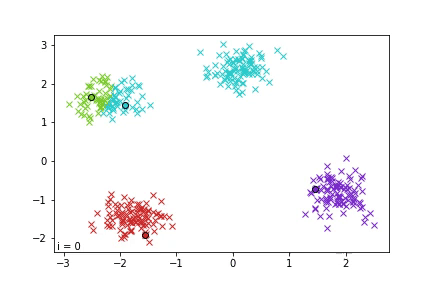This, however, means that it's terrible at recognizing clusters of different shapes. It cannot really be tweaked to fix this problem because it's the core of the algorithm, so the only recommendation we can give here is to try your best to visualize your data beforehand and see the shapes that you're aiming to cluster.

If you can't do that effectively, another indication that this may be a problem is high SEE when testing your K-Means clustering.

If that's the case and you can't fix it by removing outliers or taking similar steps, consider using a different clustering method that's better suited to different shapes of clusters (i.e. DBSCAN) and seeing if your results improve: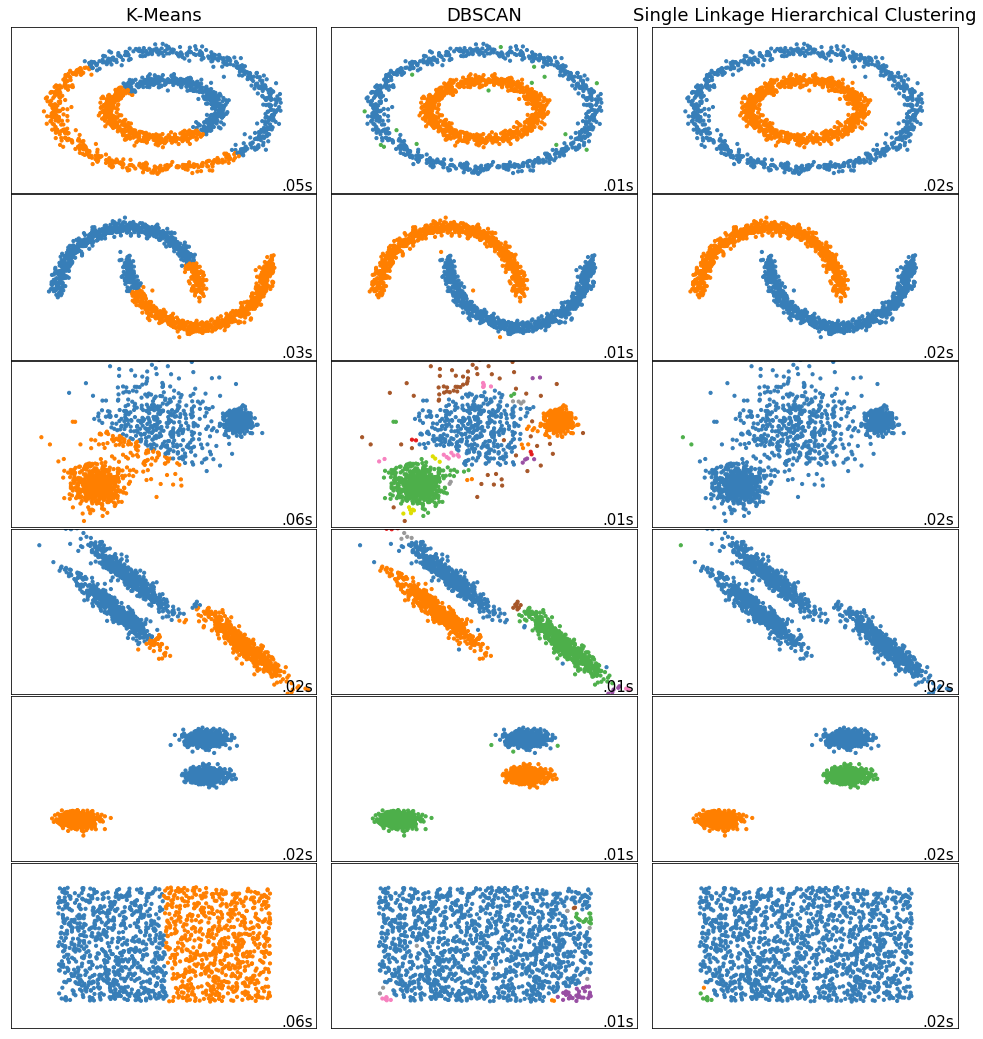The second very obvious type of dataset K-Means will have problems with is a dataset full of clusters with inconsistent sizes. If you have a big wide cluster and right beside it a tiny cluster, the tiny cluster will often be entirely swallowed by the big one.

This is because it doesn't severely negatively impact its SSE because it just slightly increases its diameter. If we somehow do end up with two centroids in these two clusters, the big cluster would likely be divided in two rather than detecting the actual existing clusters.

This is again because the SSE of a big wide cluster and a tiny one is going to be greater than the SSE of a halved big cluster. Again, as with previous sections, we recommend visualization and/or comparing results with different methods (i.e. hierarchical clustering) to determine whether this causes problems.

And the third mentioned problem is clusters of varying densities. Dense points are going to have a larger effect on the average than those who aren't as densely packed and they're going to be closer to their centroid than those that aren't as densely packed. Less dense clusters are going to have larger SSE and get broken apart and consumed into the surrounding dense clusters.

Here's an illustration of the problem of clusters with varying sizes and densities:### Variations of K-Means

There are variations of this algorithm that differ mainly in how the centroid is chosen. Here's a list of some of them:

• K-Modes - centroid is the item created by selecting the most frequent occurrence in the cluster for each attribute.
• K-Medoids - similar to a mean, but it's restricted to being an actual member of the data set, rather than just a possible value.
• K-Median - instead of the mean, we use the median or the "middle element" for each attribute to create our centroid.
• Expectation–Maximization (EM) Clustering using Gaussian Mixture Models (GMM) - detects elliptical shapes through using both a mean and a standard deviation to define membership in a cluster.

### Conclusion

We have provided an intuition behind K-Means through drawing parallels with the human experience, went through the details of how it can be implemented, various concerns we should be mindful of when implementing it, and common problems encountered while working with it. We've also mentioned similar algorithms, as well as alternative clustering algorithms for situations where K-Means falls short.

Last Updated: March 16th, 2021

## Improve your dev skills!

Get tutorials, guides, and dev jobs in your inbox.

No spam ever. Unsubscribe at any time. Read our Privacy Policy.

# Prepping for an interview?

• Improve your skills by solving one coding problem every day
• Get the solutions the next morning via email
• Practice on actual problems asked by top companies, like:

# Git Essentials

Check out this hands-on, practical guide to learning Git, with best-practices and industry-accepted standards. Stop Googling Git commands and actually learn it!

© 2013-2021 Stack Abuse. All rights reserved.